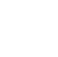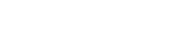# Physics Paper 2 Questions - Maranda Mocks 2021/2022 Exams

PHYSICS
Paper 2

Instructions to candidates

• This paper consists of two sections A and B
• Answer ALL the questions in sections A and B in the spaces provided.
• All workings must be clearly shown. Mathematical tables and silent electronic calculators may be used
(Take g = 10 N/kg or 10 m/s2; charge of an electron = 1.6 x 10-19 C; Planck’s constant = 6.63 x 10-34 Js)## Questions

SECTION A (25 MARKS)
Answer all the questions in the spaces provided

1. Figure 1 shows a straight conductor carrying current. Draw magnetic fields around it. (1mk)2. A ray of white light is incident on a transparent triangular glass prism.
1. State what is observed on the prism (1 mk)
2. Explain the cause for what is observed on the prism (1mk)
3. An electric kettle rated 2.5 kW, 240 V is filled with water that requires 7.2 x 105 J of energy to boil it from the room temperature. Determine the time taken to heat the water (2mks)
4.  Figure 2 represents part of the main circuit.1. Explain why it is not advisable to fix a fuse on neutral line. (1mk)
2. Explain why there are fuses of different rating in the distribution box. (1mk)
3. State the advantage of a circuit breaker over fuse when used in the fuse box (2mks)
5. Two electromagnetic waves A and B have wavelengths of 1 x 10 -9 m and 7.5 x 10-7 m respectively. State and explain which of the two waves is likely to cause cancer (2mks)
6. An optician’s test card is fixed 70 cm behind the eyes of a patient who looks into a plane mirror as shown in figure 3.Determine the distance between the eye and the image (3mks)
7. Figure 4 shows paths taken by three radiations K, J and L from a radioactive through an electric field.1. State the charge on plate Y (1mk)
2. Identify the radiations K (1mk)
3. Give a reason why L deviates more than J (1mk)
8. Figure 5 shows the image, I, formed by a concave mirror. Locate using ray diagrams the position of the object. (2mks)9. State two defects of a simple cell and how each can be minimized. (2mks)
10. A ray of light is incident on a plane surface of a transparent material at such an angle that the reflected and the refracted rays are at right angles to each other. Calculate the refractive index if the angle of incidence is 60˚. (3mks)
11. State one factor other than thickness, which determines the frequency of sound from stretched wire at room temperature. (1mk)

SECTION B: 55 MARKS
Answer all questions in this section

1.
1. Figure 6 shows a highly negatively charged cloud above a lightning arrester placed at the highest point of a storey building. Use it to answer the questions that follow;1. Indicate on the lightning arrester the charge distribution around the spikes (1mk)
2. Explain how the lightning arrester works (3mks)
2. Figure 7 shows part of the circuit containing two capacitors of 2.5μF and 3.5μF respectively.Determine the p.d across XY given that the total charge in the capacitors is 2.5 x 10-4 coulombs. (3mks)
3. Figure 8 shows a network of resistors connected to power supply of 15V of internal resistance 0.6 Ω.Determine:
1. The effective resistance of the network (2mks)
2. The voltage across 3Ω resistor (3mks)
4.  In a simple circuit consisting of a cell of e.m.f. E of internal resistance r, the potential difference across a bulb in that circuit is given as V. Given that; E=V+1r, show that; (3mks)
1/l=  R/r/E
2. Figure 9 shows a graph obtained in an experiment to investigate the relationship between stopping potential and frequency of radiation. Use it to answer the questions that follow.1. Given that ℎf=Wo + ½meV2max show that Vs= ℎf/eℎfo/e(2mks)
2. From the graph determine:
1. The threshold frequency, fo of the metal (2mks)
2. Work function of the metal in electron volts (3mks)
(Take charge of an electron = 1.6 x 10-19 C)
3.
1. Figure 10 shows a pair of U-shaped iron pieces wound with insulated wire of 15 turns and 30 turns respectively. The primary coil is connected to a p.d. of 1.0 V a.c. and has a torch bulb connected across it.1. State the observation made on the bulbs (1mk)
2. Determine the reading read from an a.c. voltmeter connected across bulb B (3mks)
2. An ideal transformer has 500 turns in the primary circuit and 2000 turns in the secondary circuit. When the primary circuit is connected to a 240 v main supply, the power delivered in the resistor in the secondary circuit is found to be 1000 W.
1. Giving a reason compare the thickness of the wires used in the primary and secondary circuit (2mks)
2. Determine the current in the circuit (2mks)
3. Determine the current in the primary circuit (4mks)
4.
1. Figure 10 shows a displacement-time graph of a wave. The velocity of the wave is 330 m/s.Determine;
1. Frequency (2mks)
2. wavelength (2mks)
2. Figure 10 shows a graph of magnification against object distance, for an object placed in front of a converging lens of a focal length 15 cm.Using the graph
1. State the effect on the size of the image when the object distance is increased from 25cm (1mk)
2. Determine the distance between the object and the mirror when the image is the same size as the object (2mks)
3. Determine the image distance when the object distance is 25cm (2mks)
3. State how longsightedness is corrected (1mk)
5.
1. Explain conductors in terms of energy band theory (2mks)
2. State the material that is used for doping an N-type semi conductor. (1mk)
3.
1. Figure 11 shows a diode. Complete the circuit to show how reverse biasing is achieved (2mks)2. What causes conduction of the diode above when it is reversed bised (1mk)
4.
1. Explain what is rectification. (1mk)
2. By use of a diagram explain full-wave rectification using two diodes (3mks)
3. In the space provided, sketch a voltage -time graph for a smoothened output for a full wave rectification (1mk)

• ✔ To read offline at any time.
• ✔ To Print at your convenience
• ✔ Share Easily with Friends / Students